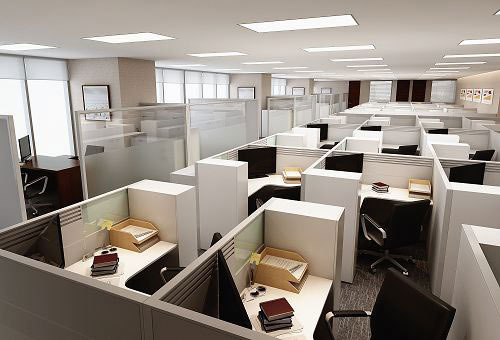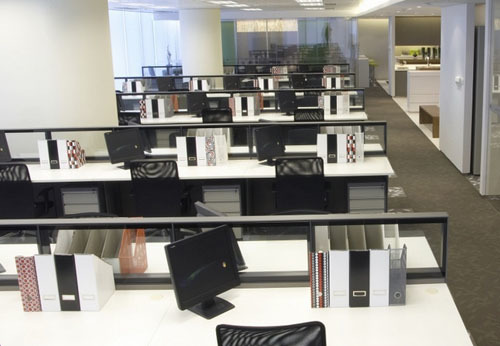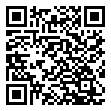|

# 办公室风水如何布局才能增旺财运?

现在不少的职场白领都非常重视办公室的风水，因为办公室风水的好坏，会直接对自己的事业、财运等运势产生不同程度的影响。那么办公室在布局的时候都应该考虑哪些风水因素呢？下面就跟着小编一起来了解吧。办公室风水讲究

1、办公室的进门设计，会影响整个办公室的格局，必须加以仔细地考量，办公室门口就像一个关卡，进门的方位、摆设、设计，会影响整个办公室的磁场，进而影响财运。大门正对着内部办公室的门，是风水上的冲，气流易直冲而进，容易引起口舌上的人事纠纷，必须在内部隔间时考虑到。

2、柜台最好摆在旺地。需考虑方便性，服务性柜台最好面对门口或进门处右边，结帐柜台则在两边旺地，如遇流年可考虑摆小盆栽，以增加人气聚财气。办公室的“财位”对事业发展有锦上添花的效果，也是每一位人士最关心的风水基准，因此风水学的确很讲究财位上的物品效应。就一般而言，财位是在进门的左前方或右前方对角线处，此处必须是很少走动之处，不能是通道，否则财运会守不住。如果右前方财位刚好是一个门，就要换找左前方财位了，有些房子因格局或设计的关系从而找不到财位，或是刚好在财位的方位是大柱子凹进来，都是风水不佳的房子，最好是运用走道隔间，造出一个财位。财位楚忌：财位上不可放置会发热的电器品；财位上不可乱堆置物品，或不加清理，布满灰尘；财位上不可放人造花、干燥花；财位上方的天花板不可有漏水，墙壁或地板油漆不可脱落或磁砖斑剥。

3、座椅后面，不可有通道，更忌讳有人走动，会心神不宁，影响工作效率。

4、办公室植栽摆设，最好是木本阔叶阳木，不要种植藤蔓类植物，容易造成纷争纠纷。

5、办公室的门不要太多，一般不要超过三门，门代表口舌是非，门太多老板主管意见容易分歧，决策难以决定。

6、职层区分方面，当然是职位越高者在越在后面。老板与主管的办公室不能在员工们的前方或正中央，一定要单独一间，不可采开放式空间。因为老板与主管是属于领导阶段，公司业务一定有其机密性，不可不加以屏敝，老板及主管的房间，宜适当或略小而雅，不可大而无当。

7、办公室前门不宜直对着后门，尤以形成直线通道，钱财流失，员工容易意见不合。当然更不可一进大门就正对着厕所的门。一般街边大楼办公室建筑都有骑楼，因此二楼处必有空间是在骑楼上方，在风水学上言，骑楼上方的房间不可当做主管决策办公室，只能供一般职员办公用，因为地板下方的骑楼，是人来人往之地道，气流很乱且杂，气场也常受干扰，因此上层楼不可当做决策场所，另外还有不少小商人，将一楼做商店用，二楼当住宅，此时要注意，二楼的厕所不可以设在一楼的老板办公室、柜台及神位的上方。

8、人尽量不要坐在房屋横梁下，坐久会伤害健康，阻碍事业发展，尤其是主管办公室和会议室，会导致意见不合、口角冲突。

9、复印机等会动的机械器具尽量放置在左方，不宜放置在老板或主管右边。通常在一个室内空间中，在分配房间的原则上，应配合“龙边”和“虎边”，也就是将办公室内部，分成左右两边，属龙的半边宜设老板办公室、业务部、财务部，其它部门就设在虎边；每间办公室的门位也要考虑，最好是设在龙边动方，事业才会兴盛发达。判断龙边的方法是：人站在室内面对开门的那一面墙，右前方是虎边，左前方是龙边，门就开在那一面墙的左侧。办公室风水如何布置增旺财运

一般而言有下列应注意事项：

1、大门正对着内部办公室的门，是风水上的冲，气流易直冲而进，容易引起口舌上的人事纠纷，必须在内部隔间时考虑到。

2、当然更不可一进大门就正对着厕所的门。

3、老板办公室一定要单独一间（套间更好），不可采开放式空间，因为公司业务一定有其机密性，不可不加以屏敝，老板及主管的房间宜适当或略小而雅，不可大而无当。

4、一般街边大楼办公室建筑都有骑楼，因此二楼处必有空间是在骑楼上方，在风水学上言，骑楼上方的房间不可当做主管决策办公室，只能供一般职员办公用，因为地板下方的骑楼，是人来人往之地道，气流很乱且杂，气场也常受干扰，因此上层楼不可当做决策场所，另外还有不少小商人，将一楼做商店用，二楼当住宅，此时要注意，二楼的厕所不可以设在一楼的老板办公室、柜台及神位的上方。

5、通常在一个室内空间中，在分配房间的原则上，应配合“龙边”和“虎边”，也就是将办公室内部，分成左右两边，属龙的半边宜设老板办公室、业务部、财务部，其它部门就设在虎边；每间办公室的门位也要考虑，最好是设在龙边动方，事业才会兴盛发达。

判断龙边的方法是：人站在室内面对开门的那一面墙，右前方是虎边，左前方是龙边，门就开在那一面墙的左侧。

6、办公室的“财位”对事业发展有锦上添花的效果，也是每一位人士最关心的风水基准，因此风水学的确很讲究财位上的物品效应。就一般而言，财位是在进门的左前方或右前方对角线处，此处必须是很少走动之处，不能是通道，否则财运会守不住。如果右前方财位刚好是一个门，就要换找左前方财位了，有些房子因格局或设计的关系从而找不到财位，或是刚好在财位的方位是大柱子凹进来，都是凤水不佳的房子，最好是运用走道隔间，造出一个财位。办公室风水布局

第一：办公桌位置

工作几年，在本职岗位上已小有成就的你，已荣升为主管，这时你要注意自己座位的后方不能是窗，就是不能为空，后方为空就意味着没有依靠，职场的路很长，这可不利于长久发展。

第二：吉祥物

吉祥物有引发和加强某一信息的作用，职场人士工作中难免遇到挫折，这时可以在个人有利的五行方位上放置相应的吉祥物，这样会使你的愿望更好更快的实现。

我们都知道左青龙右白虎，青龙位是在左边，平时在桌面的左边摆放名片、文昌塔以及一些略高的物品，可以增加青龙位的力量，会提高你的职场知名度和领导力。

第三：办公桌的整洁

白领的工作普遍比较繁忙，再忙也要注意办公桌台面的整洁，经常看到有的人办公桌上满是资料、文件、书籍，漂亮美眉们还要放些好玩的装饰物，建议增加情趣的同时也要考虑整洁的问题，桌面上的东西以少为好，清洁而有序才能提升工作效率。

第四：座位上方不宜出现横梁

除了床上方不能有横梁，办公室也一样，如果你的座位上方有梁柱，容易使工作出现障碍，尤其在与人沟通方面，多有冲突和争执。

第五：不规则的房间做办公室也会影响职场发展有些办公大厦的构造比较标新立异，导致内部会出现不规则状的房间，这样的办公室气场分布不均衡，磁场也会不稳定，容易造成人情绪的不稳定，长期在“无所适从”的状态下，难免会在工作决策中造成判断失误，影响职场发展。

第六：窗外不能有“角煞”

房间内的风水我们会比较重视，房间外也有一些风水禁忌，首先，窗外不能有“角煞”。角煞就是从室内能看见对面建筑物的锐角，角煞对人的正常能量有冲煞作用，长期在此工作会使人的精神无法集中，脑部的磁场也会被干扰，思维自然也会受到影响。

第七：办公室颜色

办公室的结构对人的影响无庸置疑，可能您有所不知，办公室的颜色也对风水有影响，东向办公室，宜以黄色作为主色，在选择办公室墙纸、沙发、装饰物时宜选用黄色系列，多用黄色可达到旺气旺财的效果。南向办公室，宜以白色来作为主色。因为白色是五行“金”的代表色。南方是火旺之地，白色是冷色调，冷色调来装饰房间可消减燥热的火气。西向办公室，宜以绿色来作为主色，绿色是木的代表色如果用这种颜色做布置，有旺财的效果，西向的办公室下午夕照强烈，绿色清淡护眼，让人有舒适感。想要生旺北向办公室的财气，应选用红色、紫色、粉红色，红紫色可增添温暖的感觉，特别是冬季会倍感温馨。

`声明：本文由入驻焦点开放平台的作者撰写，除焦点官方账号外，观点仅代表作者本人，不代表焦点立场错误信息举报电话： 400-099-0099，邮箱：jubao@vip.sohu.com，或点此进行意见反馈，或点此进行举报投诉。`A B C D E F G H J K L M N P Q R S T W X Y Z
A - B - C - D - E
• A
• 鞍山
• 安庆
• 安阳
• 安顺
• 安康
• 澳门
• B
• 北京
• 保定
• 包头
• 巴彦淖尔
• 本溪
• 蚌埠
• 亳州
• 滨州
• 北海
• 百色
• 巴中
• 毕节
• 保山
• 宝鸡
• 白银
• 巴州
• C
• 承德
• 沧州
• 长治
• 赤峰
• 朝阳
• 长春
• 常州
• 滁州
• 池州
• 长沙
• 常德
• 郴州
• 潮州
• 崇左
• 重庆
• 成都
• 楚雄
• 昌都
• 慈溪
• 常熟
• D
• 大同
• 大连
• 丹东
• 大庆
• 东营
• 德州
• 东莞
• 德阳
• 达州
• 大理
• 德宏
• 定西
• 儋州
• 东平
• E
• 鄂尔多斯
• 鄂州
• 恩施
F - G - H - I - J
• F
• 抚顺
• 阜新
• 阜阳
• 福州
• 抚州
• 佛山
• 防城港
• G
• 赣州
• 广州
• 桂林
• 贵港
• 广元
• 广安
• 贵阳
• 固原
• H
• 邯郸
• 衡水
• 呼和浩特
• 呼伦贝尔
• 葫芦岛
• 哈尔滨
• 黑河
• 淮安
• 杭州
• 湖州
• 合肥
• 淮南
• 淮北
• 黄山
• 菏泽
• 鹤壁
• 黄石
• 黄冈
• 衡阳
• 怀化
• 惠州
• 河源
• 贺州
• 河池
• 海口
• 红河
• 汉中
• 海东
• 怀来
• I
• J
• 晋中
• 锦州
• 吉林
• 鸡西
• 佳木斯
• 嘉兴
• 金华
• 景德镇
• 九江
• 吉安
• 济南
• 济宁
• 焦作
• 荆门
• 荆州
• 江门
• 揭阳
• 金昌
• 酒泉
• 嘉峪关
K - L - M - N - P
• K
• 开封
• 昆明
• 昆山
• L
• 廊坊
• 临汾
• 辽阳
• 连云港
• 丽水
• 六安
• 龙岩
• 莱芜
• 临沂
• 聊城
• 洛阳
• 漯河
• 娄底
• 柳州
• 来宾
• 泸州
• 乐山
• 六盘水
• 丽江
• 临沧
• 拉萨
• 林芝
• 兰州
• 陇南
• M
• 牡丹江
• 马鞍山
• 茂名
• 梅州
• 绵阳
• 眉山
• N
• 南京
• 南通
• 宁波
• 南平
• 宁德
• 南昌
• 南阳
• 南宁
• 内江
• 南充
• P
• 盘锦
• 莆田
• 平顶山
• 濮阳
• 攀枝花
• 普洱
• 平凉
Q - R - S - T - W
• Q
• 秦皇岛
• 齐齐哈尔
• 衢州
• 泉州
• 青岛
• 清远
• 钦州
• 黔南
• 曲靖
• 庆阳
• R
• 日照
• 日喀则
• S
• 石家庄
• 沈阳
• 双鸭山
• 绥化
• 上海
• 苏州
• 宿迁
• 绍兴
• 宿州
• 三明
• 上饶
• 三门峡
• 商丘
• 十堰
• 随州
• 邵阳
• 韶关
• 深圳
• 汕头
• 汕尾
• 三亚
• 三沙
• 遂宁
• 山南
• 商洛
• 石嘴山
• T
• 天津
• 唐山
• 太原
• 通辽
• 铁岭
• 泰州
• 台州
• 铜陵
• 泰安
• 铜仁
• 铜川
• 天水
• 天门
• W
• 乌海
• 乌兰察布
• 无锡
• 温州
• 芜湖
• 潍坊
• 威海
• 武汉
• 梧州
• 渭南
• 武威
• 吴忠
• 乌鲁木齐
X - Y - Z
• X
• 邢台
• 徐州
• 宣城
• 厦门
• 新乡
• 许昌
• 信阳
• 襄阳
• 孝感
• 咸宁
• 湘潭
• 湘西
• 西双版纳
• 西安
• 咸阳
• 西宁
• 仙桃
• 西昌
• Y
• 运城
• 营口
• 盐城
• 扬州
• 鹰潭
• 宜春
• 烟台
• 宜昌
• 岳阳
• 益阳
• 永州
• 阳江
• 云浮
• 玉林
• 宜宾
• 雅安
• 玉溪
• 延安
• 榆林
• 银川
• Z
• 张家口
• 镇江
• 舟山
• 漳州
• 淄博
• 枣庄
• 郑州
• 周口
• 驻马店
• 株洲
• 张家界
• 珠海
• 湛江
• 肇庆
• 中山
• 自贡
• 资阳
• 遵义
• 昭通
• 张掖
• 中卫

1室1厅1厨1卫1阳台

1
2
3
4
5

0
1
2

1

1

0
1
2
3报名成功，资料已提交审核A B C D E F G H J K L M N P Q R S T W X Y Z
A - B - C - D - E
• A
• 鞍山
• 安庆
• 安阳
• 安顺
• 安康
• 澳门
• B
• 北京
• 保定
• 包头
• 巴彦淖尔
• 本溪
• 蚌埠
• 亳州
• 滨州
• 北海
• 百色
• 巴中
• 毕节
• 保山
• 宝鸡
• 白银
• 巴州
• C
• 承德
• 沧州
• 长治
• 赤峰
• 朝阳
• 长春
• 常州
• 滁州
• 池州
• 长沙
• 常德
• 郴州
• 潮州
• 崇左
• 重庆
• 成都
• 楚雄
• 昌都
• 慈溪
• 常熟
• D
• 大同
• 大连
• 丹东
• 大庆
• 东营
• 德州
• 东莞
• 德阳
• 达州
• 大理
• 德宏
• 定西
• 儋州
• 东平
• E
• 鄂尔多斯
• 鄂州
• 恩施
F - G - H - I - J
• F
• 抚顺
• 阜新
• 阜阳
• 福州
• 抚州
• 佛山
• 防城港
• G
• 赣州
• 广州
• 桂林
• 贵港
• 广元
• 广安
• 贵阳
• 固原
• H
• 邯郸
• 衡水
• 呼和浩特
• 呼伦贝尔
• 葫芦岛
• 哈尔滨
• 黑河
• 淮安
• 杭州
• 湖州
• 合肥
• 淮南
• 淮北
• 黄山
• 菏泽
• 鹤壁
• 黄石
• 黄冈
• 衡阳
• 怀化
• 惠州
• 河源
• 贺州
• 河池
• 海口
• 红河
• 汉中
• 海东
• 怀来
• I
• J
• 晋中
• 锦州
• 吉林
• 鸡西
• 佳木斯
• 嘉兴
• 金华
• 景德镇
• 九江
• 吉安
• 济南
• 济宁
• 焦作
• 荆门
• 荆州
• 江门
• 揭阳
• 金昌
• 酒泉
• 嘉峪关
K - L - M - N - P
• K
• 开封
• 昆明
• 昆山
• L
• 廊坊
• 临汾
• 辽阳
• 连云港
• 丽水
• 六安
• 龙岩
• 莱芜
• 临沂
• 聊城
• 洛阳
• 漯河
• 娄底
• 柳州
• 来宾
• 泸州
• 乐山
• 六盘水
• 丽江
• 临沧
• 拉萨
• 林芝
• 兰州
• 陇南
• M
• 牡丹江
• 马鞍山
• 茂名
• 梅州
• 绵阳
• 眉山
• N
• 南京
• 南通
• 宁波
• 南平
• 宁德
• 南昌
• 南阳
• 南宁
• 内江
• 南充
• P
• 盘锦
• 莆田
• 平顶山
• 濮阳
• 攀枝花
• 普洱
• 平凉
Q - R - S - T - W
• Q
• 秦皇岛
• 齐齐哈尔
• 衢州
• 泉州
• 青岛
• 清远
• 钦州
• 黔南
• 曲靖
• 庆阳
• R
• 日照
• 日喀则
• S
• 石家庄
• 沈阳
• 双鸭山
• 绥化
• 上海
• 苏州
• 宿迁
• 绍兴
• 宿州
• 三明
• 上饶
• 三门峡
• 商丘
• 十堰
• 随州
• 邵阳
• 韶关
• 深圳
• 汕头
• 汕尾
• 三亚
• 三沙
• 遂宁
• 山南
• 商洛
• 石嘴山
• T
• 天津
• 唐山
• 太原
• 通辽
• 铁岭
• 泰州
• 台州
• 铜陵
• 泰安
• 铜仁
• 铜川
• 天水
• 天门
• W
• 乌海
• 乌兰察布
• 无锡
• 温州
• 芜湖
• 潍坊
• 威海
• 武汉
• 梧州
• 渭南
• 武威
• 吴忠
• 乌鲁木齐
X - Y - Z
• X
• 邢台
• 徐州
• 宣城
• 厦门
• 新乡
• 许昌
• 信阳
• 襄阳
• 孝感
• 咸宁
• 湘潭
• 湘西
• 西双版纳
• 西安
• 咸阳
• 西宁
• 仙桃
• 西昌
• Y
• 运城
• 营口
• 盐城
• 扬州
• 鹰潭
• 宜春
• 烟台
• 宜昌
• 岳阳
• 益阳
• 永州
• 阳江
• 云浮
• 玉林
• 宜宾
• 雅安
• 玉溪
• 延安
• 榆林
• 银川
• Z
• 张家口
• 镇江
• 舟山
• 漳州
• 淄博
• 枣庄
• 郑州
• 周口
• 驻马店
• 株洲
• 张家界
• 珠海
• 湛江
• 肇庆
• 中山
• 自贡
• 资阳
• 遵义
• 昭通
• 张掖
• 中卫• 手机• 分享
• 设计
免费设计
• 计算器
装修计算器
• 入驻
合作入驻
• 联系
联系我们
• 置顶
返回顶部